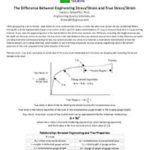Think about pulling a bar in tension. Load divided by cross-sectional area is force, or stress. But what cross section are you considering? Before starting that pull, the bar had a known cross-section of, let’s say, 0.5″ wide x metal thickness. It’s easy to measure these, since it is your starting material. At any load, the engineering stress is the load divided by this initial cross-sectional area. While you are pulling, the length increases, but the width and thickness shrink. At any load, the true stress is the load divided by the cross-sectional area at that instant. Unless thickness and width are being monitored continuously during the test, you cannot calculate true stress. It is, however, a much better representation of how the material behaves as it is being deformed, which explains its use in forming simulations. In circle grid analysis, engineering strain is the percent expansion of the circle compared to the initial diameter of the circle. The relationships between engineering values and true values are:

σ = s (1+e)         ε = ln (1+e)

Where “s” and “e” are the engineering stress and strain, respectively, and “ σ ” and “ ε ” are the true stress and strain, respectively.